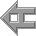The exposition continues with the study of "Phase Shifts",(this might well have preceeded the discussion of the effects of sets of parallels layered one above the other since now it will become clearer why this is stricly speaking incorrect. This was not done because the complexity of the subject was sufficient but now we can retrospectively modify it). The drawing of one line of sine curves slightly shifted to the right illustrates this and it is obvious in "Phase Shifts" fig.129, where the lines are alternately filled in to create a visual three-dimensional twist, a torsion: in phase, when the two lines coincide and out of phase when they depart from coïncidence. Such phase shifts are exquisitely demonstrated in moirés where the double image slightly shifted creates brillant more complex interferences than one could have conceived.
'Using two pencils or felt pens taped together with a small interval cover the complete sheet with a wave pattern starting from a single trace. Note the serial modification of curves leading to a surface showing a progressive deformation.---/'

Fig.127. Two pens taped together tracing a sine curve of decreasing amplitude illustrate a simple phase shift.

Fig.128. Five, free-hand drawn sine curves out of phase(drawn with two pens taped together). This, to use a musical example, mirrors the form of a rondo when the theme is repeated a moment later.

Fig.129. Blackening the above Fig.128. in alternate paths creates a three-dimensionnal torsion.

'---/Using different colours so that both elements can be distinguished compare these results with the repeated curves made in 4A.(non-parallel at uniform intervals). Superimpose these. Consider the fundamental contraction between the curvature and the notion of parallelism in view of the theoretical impossibility of the straight line parallel, itself theoretically unrealizable except within strictly limited fields.'

Fig.129bis. Sine curves out of phase superimposed on ellipses (free-hand drawn with two pens taped together)

This poses a question about the nature of optical reality opposed to that of physical reality, reason trying hard to impose categories which do not apply. Refer back to the previous page 'the notion of parallelism in view of the theoretical impossibility of straight line parallels, itself theoretically unrealizable except within strictly limited fields.' What do we mean by parallel? The obvious answer is that a straight line is parallel to another if perpendicular lines between them are equally far apart. However, even in cases of straight line parallels drawn in the space of a convergent field, there are optical distortions. For a graphic illustration of this see page 58 where straight line parallels are drawn within a rectilinear and a converging space. The straight lines are apparently curved around the centre of the convergence. Even in this most simple case ambiguities arise. The question of curved line parallels is even more complex. What could one mean by curved line parallels? One would say, as a first approximation, that a system of sine waves drawn one below the other at a regular distance should be parallel. However, it is obvious on inspection that this is not the case.

Fig.130. System of sine waves drawn one below the other at a regular distance. The perpendiculars drawn vertically do not equal those drawn on the curve. If the curve is continuous these vertical lines are only singularities within the infinity of possible curves. Thus the concept of curved line parallels is questionable.

Fig.131. 'Waves' shapes.
Going back to waves: in physical fact the shape of each wave varies slightly until the waves interfere and finally cross each other forming, so to speak, a kink, and they collapse, running out on the shore or even in mid-sea.
It is an appreciation of this contradiction between optical and physical reality which lends such sophistication to Hayter's drawing, painting and etching of wave movements.back